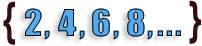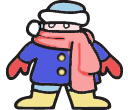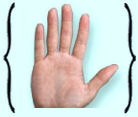# Introductions to Sets

## Set Theory Lesson and Examples: Introductions to Sets

Use the following examples and interactive exercises to learn about Introductions to Sets.Example 1: Kyesha was in math class with her friend Angie. She whispered to Angie that she had just bought a set of winter clothes. The outerwear collection includes a coat, a hat, a scarf, gloves, and boots. Their teacher, Mrs. Glosser, overheard the conversation and asked them: What is a set?

Solution: Luckily for Kyesha and Angie, their classmate Eduardo had a math dictionary with him! He quickly looked up the word "set" and defined it for the class as shown below.

set is a collection of objects that have something in common or follow a rule. The objects in the set are called its elements. Set notation uses curly braces, with elements separated by commas. So the set of outwear for Kyesha would be listed as follows:

A = {coat, hat, scarf, gloves, boots}, where A is the name of the set, and the braces indicate that the objects written between them belong to the set.

Every object in a set is unique: The same object cannot be included in the set more than once.

Let's look at some more examples of sets.Example 2: What is the set of all fingers?

Solution: P = {thumb, index, middle, ring, little}

Note that there are others names for these fingers: The index finger is commonly referred to as the pointer finger; the ring finger is also known as the fourth finger, and the little finger is often referred to as the pinky. Thus, we could have listed the set of fingers as:

P = {thumb, pointer, middle, fourth, pinky}Example 3: What is the set of all even whole numbers between 0 and 10?

Solution: Q = {2, 4, 6, 8}  Note that the use of the word between means that the range of numbers given is not inclusive. As a result, the numbers 0 and 10 are not listed as elements in this set.Example 4: Eduardo was in art class when the teacher wrote this on the chalkboard: In fine arts, primary colors are sets of colors that can be combined to make a useful range of colors. Then she asked the class: What is the set of primary colors?

Solution: Eduardo answered: red, blue and yellow. Angie answered: We can use set notation to list the set of all primary colors.Kyesha went to the chalkboard and wrote:

X = {red, blue, yellow}

The teacher said: Good work everyone. This is a nice combination of art and math!

In examples 1 through 4, each set had a different number of elements, and each element within a set was unique. In these examples, certain conventions were used.

The following conventions are used with sets:

• Capital letters are used to denote sets.
• Lowercase letters are used to denote elements of sets.
• Curly braces { } denote a list of elements in a set.

So for examples 1 through 4, we listed the sets as follows:

1. A = {coat, hat, scarf, gloves, boots}
2. P = {thumb, index, middle, ring, little}
3. Q = {2, 4, 6, 8}
4. X = {red, blue, yellow}

These sets have been listed with roster notation. Roster notation is a list of elements, separated by commas, enclosed in curly braces. The curly braces are used to indicate that the elements written between them belong to that set. Let's look at some more examples of sets listed with roster notation.

Example 5: Let R be the set of all vowels in the English alphabet.

Solution: R = {a, e, i, o, u}

Example 6: Let G be the set of all whole numbers less than ten.

Solution: G = {0, 1, 2, 3, 4, 5, 6, 7, 8, 9}

Example 7: Let T be the set of all days in a week.

Solution: T = {Monday, Tuesday, Wednesday, Thursday, Friday, Saturday, Sunday}

Example 8: Let X be the set of odd numbers less than 12.

Solution: X = {1, 3, 5, 7, 9, 11}

Example 9: Let Y be the set of all continents of the world.

Solution: Y = {Asia, Africa, North America, South America, Antarctica, Europe, Australia}

There are times when it is not practical to list all the elements of a set. In this case, it is better to describe the set. The rule that the elements follow can be given in the braces. For example,:

R = {vowels} means Let R be the set of all vowels in the English alphabet.

This is especially useful when working with large sets, as shown below.

 A = {types of triangles}G = {letters in the English alphabet} J = {prime numbers less than 100} M = {state capitals in the US}

When describing a set, It is not necessary to list every element in that set. Thus, there are two methods for indicating a set of objects: 1) listing the elements and 2) describing the elements. We will distinguish between these two methods in examples 10 and 11 below.

Example 10: What is the set of all letters in the English alphabet?

Listing Elements: D = {a, b, c, d, e, f, g, h, i, j, k, l, m, n, o, p, q, r, s, t, u, v, w, x, y, z}

Describing Elements: D = {letters in the English alphabet}

Example 11: What is the set of all states in the Unites States?

Solution: R = {all states in the US}

In example 10, set D has 26 elements, so it is easier to describe its elements than to list them. Similarly, in example 11, set R has 50 elements, so it is easier to describe its elements.

Summary: A set is a collection of objects that have something in common or follow a rule. The objects in the set are called its elements. Curly braces are used to indicate that the objects written between them belong to a set. Every object in a set is unique. It is not necessary to list every object in the set. Instead, the rule that the objects follow can be given in the braces. We can define a set by listing its elements or by describing its elements. The latter method is useful when working with large sets.